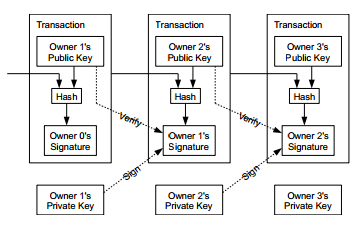Private key

Overview
One of the key pairs in public key cryptography is the private key.Private key was chosen randomly integer (256 bit) from between 1 to p.
"p" is「2^256 - 2^32 - 2^9 - 2^8 - 2^7 - 2^6 - 2^4 - 1」.
This is the order p of the elliptic curve cryptography parameter(SECp 256 k 1) used in Bitcoin.

Private key code
Let's actually generate a private key

Decode

```code_strings = {16: '0123456789abcdef'}

def decode_privkey(priv):
result = 0
while len(string) > 0:
result *= int(16)
result += code_strings[int(16)].find(priv)
prive = priv[1:]
return result
```

Decode_privkey function is function of convert hex to decimal.
Calculate one each from the beginning of the argument.。
Convert to the corresponded value with code_strings.
Find addition of converted value and previous value,and move to next value.

Random number generation

```def random_key():
entropy = str(os.urandom(32))+ str(random.randrange(2**256)) + str(int(time.time() * 1000000))
binary_data = entropy if isinstance(entropy, bytes) else bytes(entropy, 'utf-8')
bin_sha256 = hashlib.sha256(binary_data).digest()
if isinstance(bin_sha256, str):
return bin_sha256
return ''.join('{:02x}'.format(y) for y in bin_sha256)
```

"random_key" function generate random number of high entropy.
os.urandom generate random n"ｔime.time ()" get epoch seconds of the current time.
umber of 32 bit.
"random.randrange(2**256)" generate one random number from between 0 to 2^256.
"ｔime.time ()" get epoch seconds of the current time.
Checks whether type of "entropy" is bytes, and convert it to bytes if not.
Get 256 bytes of data with SHA 256.

Private key generation

```N = 115792089237316195423570985008687907852837564279074904382605163141518161494337

valid_private_key = False
while not valid_private_key:
private_key = random_key()
decoded_private_key = decode_privkey(private_key)
valid_private_key = 0 < decoded_private_key < N

print (private_key)
```

Ｎ= 2^256(to be accurate ,N = 2^256 - 2^32 - 2^9 - 2^8 - 2^7 - 2^6 - 2^4 - 1).
Random number generated by "random_key ()" and check whether it's between 0 and N.